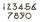Diophantus

We know little about this Greek mathematician from Alexandria, except that he lived around 3rd century A.D. Thanks to an admirer of his, who described his life by means of an algebraic riddle, we know at least something about his life.
Diophantus's youth lasted 1/6 of his life. He had his first beard in the next 1/12 of his life. At the end of the following 1/7 of his life Diophantus got married. Five years from then his son was born. His son lived exactly 1/2 of Diophantus's life. Diophantus died 4 years after the death of his son.

How long did Diophantus live? [Diophantus epitaf]

Result

x =  84

Solution:1/6* x + 1/12 * x + 1/7 * x + 5 + 1/2 * x + 4 = x

9x = 756

x = 84

Calculated by our simple equation calculator.

Leave us a comment of example and its solution (i.e. if it is still somewhat unclear...):Be the first to comment!To solve this verbal math problem are needed these knowledge from mathematics:

Need help calculate sum, simplify or multiply fractions? Try our fraction calculator. Do you have a linear equation or system of equations and looking for its solution? Or do you have quadratic equation?

Next similar examples:

1. Dropped sheetsThree consecutive sheets dropped from the book. The sum of the numbers on the pages of the dropped sheets is 273. What number has the last page of the dropped sheets?
2. SymbolsIf 2*3 = 60 ; 3*4 = 120 and 4*5 = 200, what is 2*5?
3. Fraction and a decimalWrite as a fraction and a decimal. One and two plus three and five hundredths
4. NumberWhat number I think? The third is 6 and half is 2 more than the its quarter.
5. One-third 2One-third of the people in a barangay petitioned the council to allow them to plant in vacant lots and another 1/5 of the people petitioned to have a regular garbage collection. What FRACTION of the barangay population made the petition?
6. NormThree workers planted 3555 seedlings of tomatoes in one dey. First worked at the standard norm, the second planted 120 seedlings more and the third 135 seedlings more than the first worker. How many seedlings were standard norm?3 3/4 + 2 3/5 + 5 1/2 Show your solution.
8. NumberI think the number. If I add to its third seven I get same as when to its quarter add 8. Which is the number?
9. EqnSolve equation with fractions: 2x/3-50=40+x/4
10. Sum of fractionsWhat is the sum of 2/3+3/5?
11. Unknown number 6Determine x if 1/6 of x is equal to 2/5 of the number 24.
12. WithdrawalIf I withdrew 2/5 of my total savings and spent 7/10 of that amount. What fraction do I have in left in my savings?
13. Evaluate expressionEvaluate expression using BODMAS rule: 1 1/4+1 1/5÷3/5-5/8
14. Grandmother and grandfatherGrandmother baked cakes. Grandfather ate half, then quarter of the rest ate Peter and Paul ate half of rest. For parents left 6 cakes. How many cakes maked the grandmother?
15. Unknown number 11That number increased by three equals three times itself?
16. Double 5Peter was thinking of a number. Peter doubles it and gets an answer of 8.6. What was the original number?
17. Fractions 3Calculate 1/9 of 27: